# Virial pressure

(diff) ← Older revision | Latest revision (diff) | Newer revision → (diff)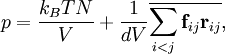$p = \frac{ k_B T N}{V} + \frac{ 1 }{ d V } \overline{ \sum_{i
where one can recognize an ideal term and another one due to the virial. The underline is an average, which would be a time average in molecular dynamics, or ensamble average in Monte Carlo;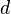$d$ is the dimension of the system (3 in actual world).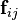${\mathbf f}_{ij}$ is the force on particle$i$ exerted by particle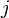$j$, and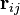${\mathbf r}_{ij}$ is the vector going from$i$ to$j$: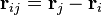${\mathbf r}_{ij} = {\mathbf r}_j - {\mathbf r}_i$.